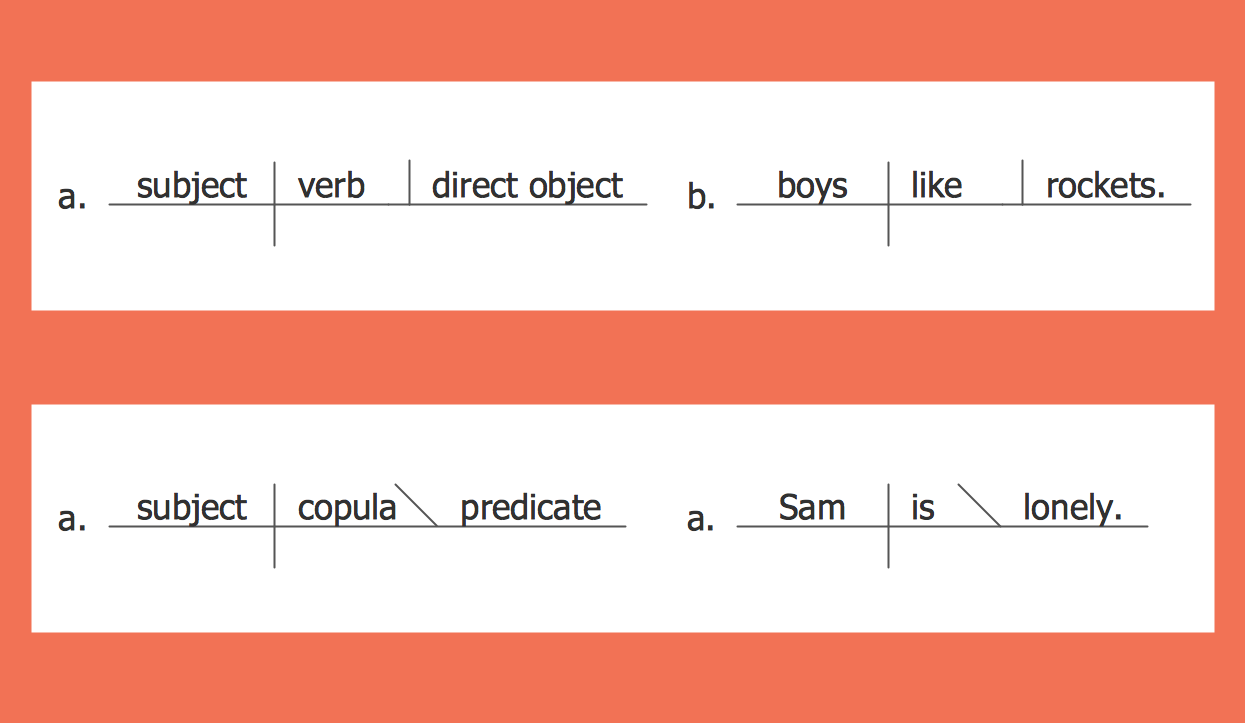ConceptDraw Samples | Science and education — Language learning

# Language Learning

Samples of sentence diagrams are created with ConceptDraw DIAGRAM diagramming and vector drawing software enhanced with Language Learning solution from ConceptDraw Solution Park.

ConceptDraw DIAGRAM provides export of vector graphic multipage documents into multiple file formats: vector graphics (SVG, EMF, EPS), bitmap graphics (PNG, JPEG, GIF, BMP, TIFF), web documents (HTML, PDF), PowerPoint presentations (PPT), Adobe Flash (SWF).

## Tutorials and Solutions:

Video Tutorials: ConceptDraw Solution Park

Solutions: Language Learning for ConceptDraw DIAGRAM

## Sample 1: Sentence Diagram — Compound

Language Learning sample: Compound sentence.

This example is created with ConceptDraw DIAGRAM diagramming and vector drawing software enhanced with Language Learning solution from ConceptDraw Solution Park.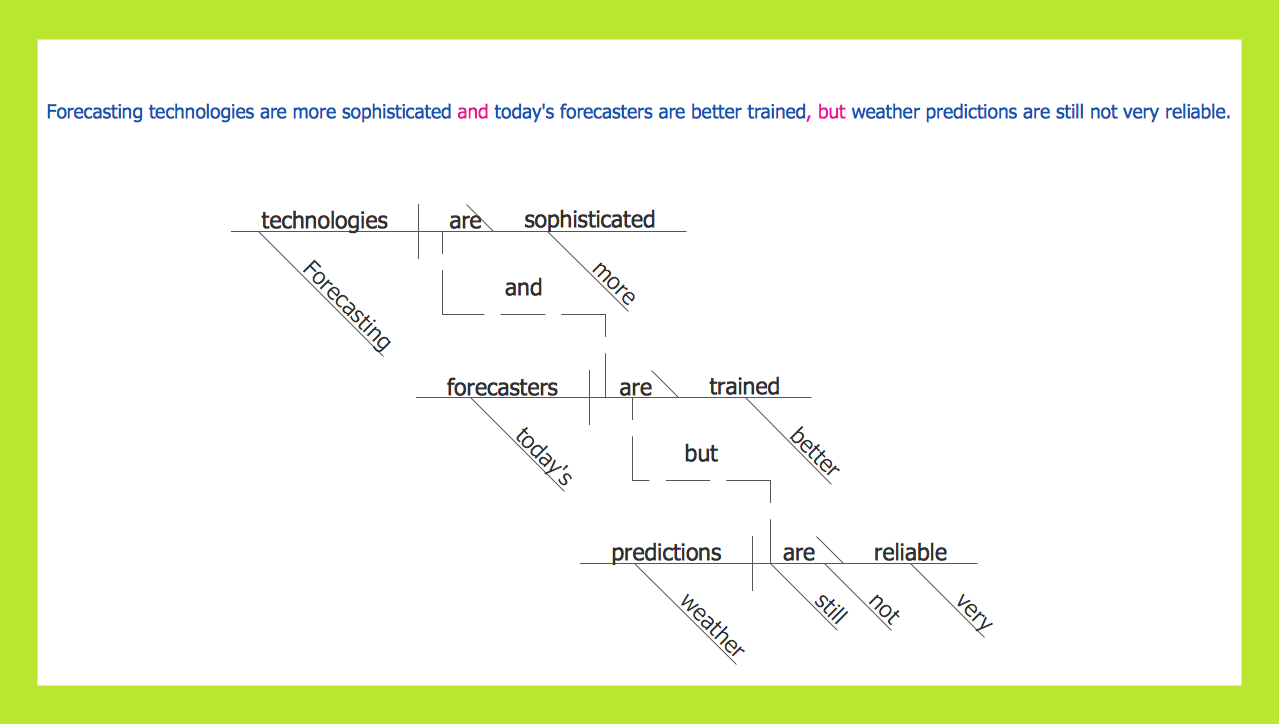## Sample 2: Sentence Diagram — Compound Predicate with One Direct Object

Language Learning sample: Compound predicate with one direct object.

This example is created with ConceptDraw DIAGRAM diagramming and vector drawing software enhanced with Language Learning solution from ConceptDraw Solution Park.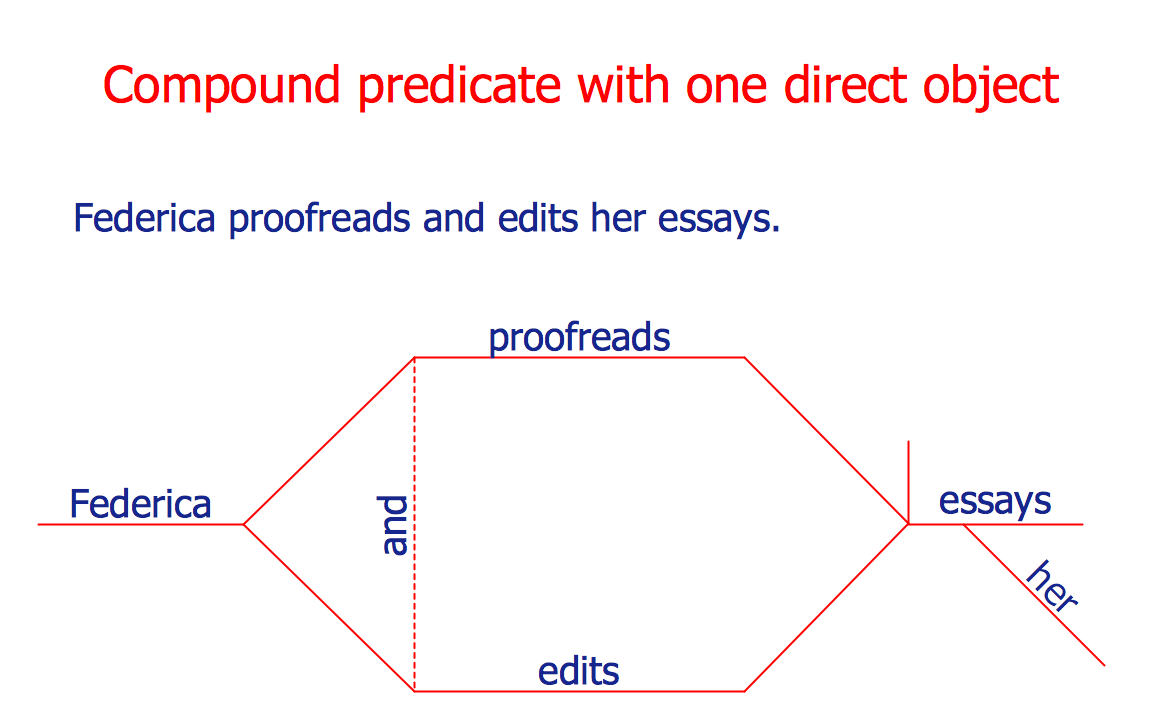## Sample 3: Sentence Diagram

Language Learning sample: Sentence diagram.

This example is created with ConceptDraw DIAGRAM diagramming and vector drawing software enhanced with Language Learning solution from ConceptDraw Solution Park.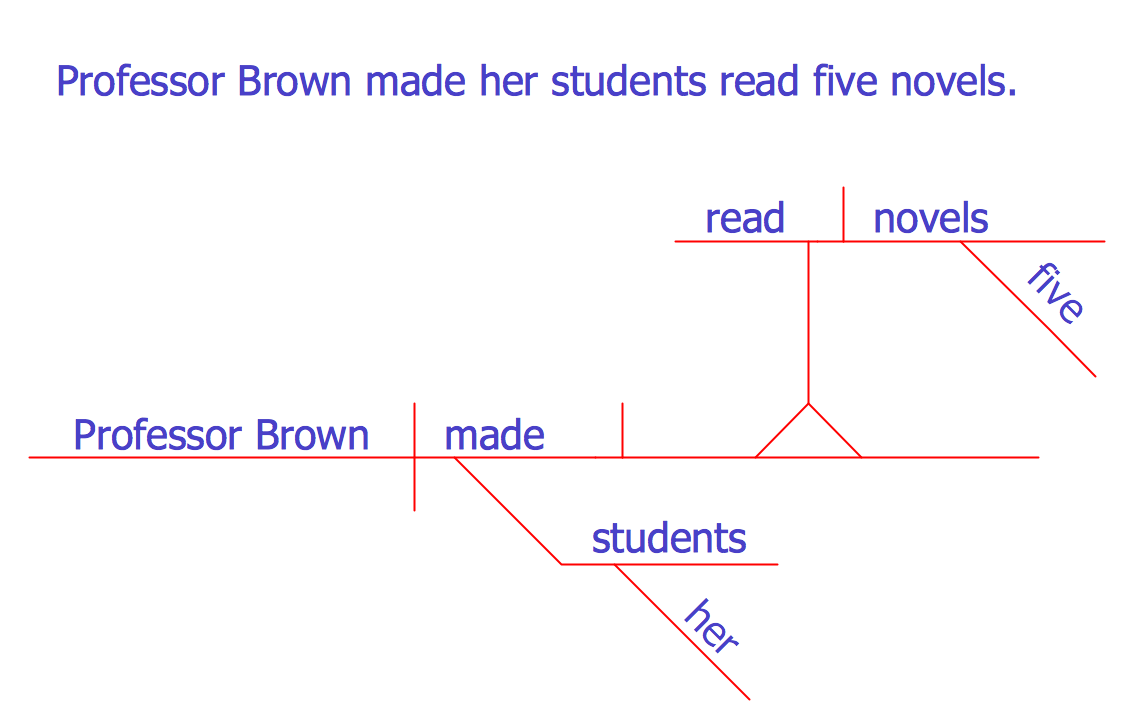## Sample 4: Sentence Diagrammer — Reed Kellogg Diagram

Language Learning sample: Reed Kellogg diagram.

This example is created with ConceptDraw DIAGRAM diagramming and vector drawing software enhanced with Language Learning solution from ConceptDraw Solution Park.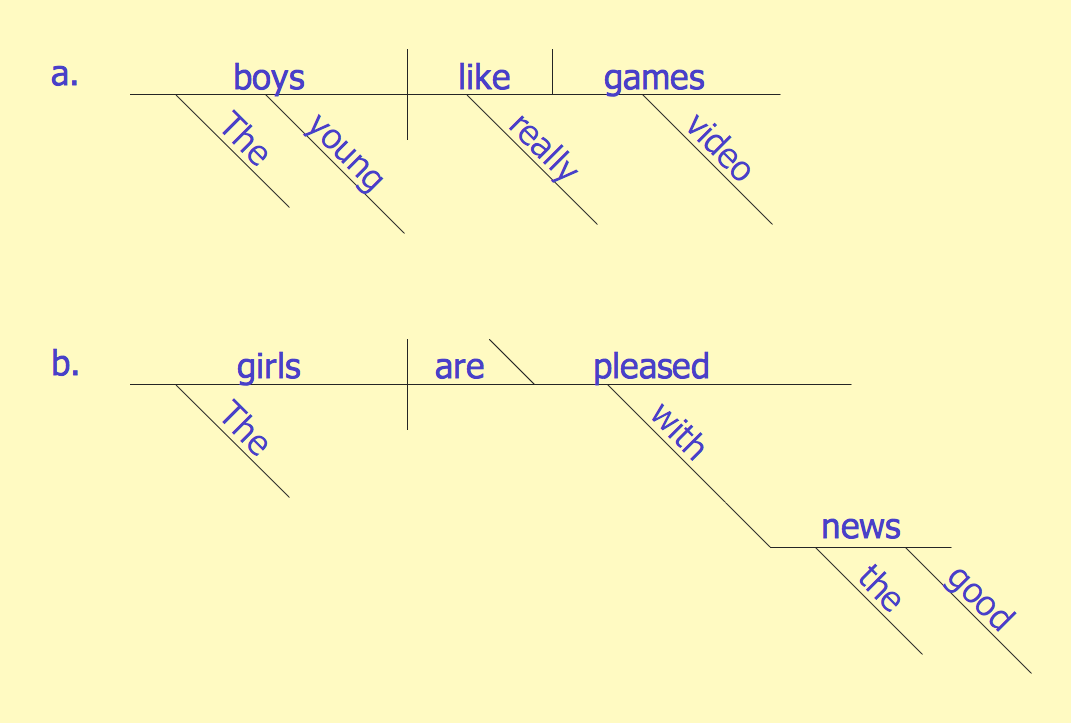## Sample 5: The Reed Kellogg System Basic Schemata

Language Learning sample: The Reed Kellogg system basic schemata.

This example is created with ConceptDraw DIAGRAM diagramming and vector drawing software enhanced with Language Learning solution from ConceptDraw Solution Park.